## How to Calculate and Solve for Escape Velocity | The Calculator Encyclopedia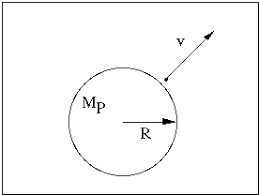The image above represents the escape velocity.

To compute the escape velocity of a field, two essential parameters are needed and the parameters are acceleration due to gravity (g) and radius (r).

The formula for calculating the escape velocity:

V = √(2gR)

Where;
V = Escape velocity
g = Acceleration due to gravity

Let’s solve an example;
Find the escape velocity of a field when the acceleration due to gravity is 12 and the radius is 24 cm.

This implies that;
g = Acceleration due to gravity = 12
r = Radius = 24 cm

V = √(2gR)
V = √(2 x 12 x 24)
V = √(576)
V = 24

Therefore, the escape velocity is 24 m/s.

## How to Calculate and Solve for Gravitational Potential | The Calculator Encyclopedia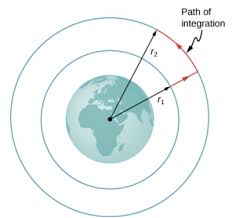The image above represents the Gravitational potential.

To compute the gravitational potential of a field, two essential parameters are needed which are mass (m) and radius (r).

The formula for calculating the gravitational potential;

V = (Gm) / r

Where;
V = Gravitational potential
m = Mass

Let’s solve an example;
Find the gravitational potential of a field when the mass is 14 cm with a radius of 9 cm.

This implies that;
m = Mass = 14 cm
r = Radius = 9 cm

V = (Gm) / r
V = (6.67 x 10-11 x 14) / 9
V = 1.0375e-10 / 9
V = 1.0375e-10

Therefore, the gravitational potential is 1.0375e-10 Volts (V).

## How to Calculate and Solve for Gravitational Force | The Calculator Encyclopedia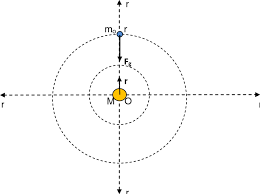The image above represents the gravitational force.

To compute the gravitational force of a field, three parameters are needed and this parameters are mass (m1), mass (m2) and radius between the masses (R).

The formula for calculating the gravitational force:

F = Gm1m2 /

Where;
F = Gravitational force
m1 = Mass 1
m2 = Mass 2
r = Radius between the masses

Let’s solve an example;
Find the gravitational force of a field when the mass 1 is 8 cm, mass 2 is 10 cm and the radius between masses is 14 cm.

This implies that;
m1 = Mass 1 = 8 cm
m2 = Mass 2 = 10 cm
r = Radius between the masses = 14 cm

F = Gm1m2 /
F = (6.67 x 10-11 x 8 x 10) / 196
F = 5.336e-9 / 196
F = 2.722e-11

Therefore, the gravitational force is 2.722e-11 Newton (N).

## How to Calculate and Solve for the Area of an Ellipsoid | The Calculator Encyclopedia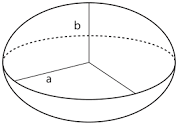The image above is an ellipsoid.

To compute the area of an ellipsoid, three essential parameters are needed and this parameters are axis (a)axis (b) and axis (c).

The formula for calculating the area of an ellipsoid:

A = 4π((ab)1.6 + (ac)1.6 + (bc)1.63)1 / 1.6

Where;
A = Area of the ellipsoid
a = Axis of the ellipsoid
b = Axis of the ellipsoid
c = Axis of the ellipsoid

Let’s solve an example;
Find the the area of an ellipsoid when the axis (a) of the ellipsoid is 12 cm, axis (b) of the ellipsoid is 6 cm and axis (c) of the ellipsoid is 2 cm.

This implies that;
a = Axis of the ellipsoid = 12 cm
b = Axis of the ellipsoid = 6 cm
c = Axis of the ellipsoid = 2 cm

A = 4π((ab)1.6 + (ac)1.6 + (bc)1.63)1 / 1.6
A = 4π(((12)(6))1.6 + ((12)(2))1.6 + ((6)(2))1.63)1 / 1.6
A = 4π(((72)1.6 + (24)1.6 + (12)1.6)3)1 / 1.6
A = 4π(((936.98) + (161.56) + (53.29))3)1 / 1.6
A = 4π((1151.84)3)1 / 1.6
A = 4π(383.946)1 / 1.6
A = 4π(383.946)0.625
A = 4π(41.2258)
A = (12.566)(41.2258)
A = 518.059

Therefore, the area of the ellipsoid is 518.059 cm2.

## How to Calculate and Solve for the Axis and Volume of an Ellipsoid | The Calculator EncyclopediaThe image above is an ellipsoid.

To compute the volume of an ellipsoid, three essential parameters are needed and this parameters are axis (a)axis (b) and axis (c).

The formula for calculating the volume of an ellipsoid:

V = 4πabc3

Where;

V = Volume of the ellipsoid
a = Axis of the ellipsoid
b = Axis of the ellipsoid
c = Axis of the ellipsoid

Let’s solve an example;
Given that the axis of the ellipsoid (a) is 9 cm and axis of the ellipsoid (b) is 11 cm with the axis (c) of 14 cm. Find the volume of the ellipsoid?

This implies that;
a = Axis of the ellipsoid = 9 cm
b = Axis of the ellipsoid = 11 cm
c = Axis of the ellipsoid = 14 cm

V = 4πabc3
V = 4π(9 x 11 x 14)3
V = 4π(1386)3
V = (12.56)(1386) / 3
V = (17416.98967) / 3
V = 5805.66

Therefore, the volume of the ellipsoid is 5805.66 cm3.

Calculating the Axis (a) of an Ellipsoid using the Volume of the Ellipsoid, Axis (b) of the Ellipsoid and Axis (c) of the Ellipsoid.

a = V3 / 4πbc

Where;
a = Axis of the ellipsoid
V = Volume of the ellipsoid
b = Axis of the ellipsoid
c = Axis of the ellipsoid

Let’s solve an example;
Find the axis (a) of an ellipsoid when the volume of the ellipsoid is 280 cm3 with an axis (b) of 18 cm and axis (c) of 8 cm.

This implies that;
V = Volume of the ellipsoid = 280 cm3
b = Axis of the ellipsoid = 18 cm
c = Axis of the ellipsoid = 8 cm

a = V3 / 4πbc
a = 280 x 3 / (12.566)(18 x 8)
a = 840 / (12.566)(144)
a = 840 / 1809.504
a = 0.46

Therefore, the axis (a) of the ellipsoid is 0.46 cm.

## How to Calculate and Solve for the Radius, Height and Surface Area of a Spherical Segment | The Calculator Encyclopedia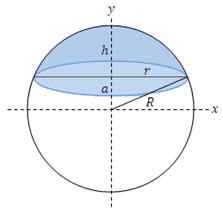The image above is a spherical segment.

To compute the surface area of a spherical segment requires two essential parameters which are the radius of the sphere (R) and the height (h).

The formula for calculating the surface area of the spherical segment:

A = 2πRh

Where;
A = Surface area of the spherical segment
R = Radius of the sphere
h = Height of the spherical segment

Let’s solve an example;
Find the surface area of a spherical segment when the radius of the sphere is 12 cm and the height is 16 cm.

This implies that;
R = Radius of the sphere = 12 cm
h = Height of the spherical segment = 16 cm

A = 2πRh
A = 2π (12 x 16)
A = 2π (192)
A = 6.28 (192)
A = 1206.37

Therefore, the surface area of the spherical segment is 1206.37 cm2.

Calculating the Radius of the Sphere using the Surface Area of the Spherical Segment and the Height.

R = A / 2πh

Where;
R = Radius of the sphere
A = Surface area of the spherical segment
h = Height of the spherical segment

Let’s solve an example;
Find the radius of a sphere with a surface area of 300 cm2 and a height of 12 cm.

This implies that;
A = Surface area of the spherical segment = 300 cm2
h = Height of the spherical segment = 12 cm

R = A / 2πh
R = 300 / 2 x π x 12
R = 300 / 75.41
R = 3.978

Therefore, the radius of the sphere is 3.978 cm.

## How to Calculate and Solve for the Volume of a Spherical Segment | Nickzom Calculator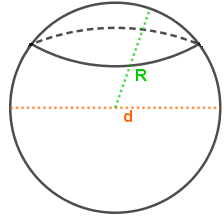The image above is a spherical segment.

To compute the volume of a spherical segment requires three essential parameters which are the radius of the spherical segment base (r1), radius of the spherical segment (r2) and height (h).

The formula for calculating the volume of the spherical segment:

V = πh(3r1² + 3r2² + h²)6

Where;
V = Volume of the spherical cap
r1 = Radius of the spherical segment base
r2 = Radius of the spherical segment base
h = Height of the spherical segment

Let’s solve an example;
Find the volume of a spherical segment when the radius of the spherical segment base (r1) is 7 cm, radius of the spherical segment base (r2) is 9 cm and a height of 20 cm.

This implies that;
r1 = Radius of the spherical segment base = 7 cm
r2 = Radius of the spherical segment base = 9 cm
h = Height of the spherical segment = 20 cm

V = πh(3r1² + 3r2² + h²)6
V = π x 20(3 x 7² + 3 x 9² + 20²)6
V = π x 20(3 x 49 + 3 x 81 + 400)6
V = π x 20(147 + 243 + 400)6
V = π x 20(790)6
V = π x 158006
V = 49643.66
V = 8273.9

Therefore, the volume of the spherical segment is 8273.9 cm3.

## How to Calculate the Radius, Height and Curved Surface Area of a Spherical Cap | The Calculator Encyclopedia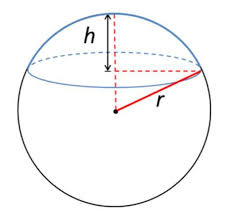The image above is a spherical cap.

To compute the curved surface area of a spherical cap requires two essential parameters which are the radius of the base of the cap (a) and the height (h).

The formula for calculating the curved surface area of the spherical cap:

A = π(a² + h²)

Where;
A = Curved surface area of the spherical cap
a = Radius of the base of the cap
h = Height of the spherical cap

Let’s solve an example;
Find the curved surface area of a spherical cap with radius of the base 7 cm and the height of 13 cm.

This implies that;
a = Radius of the base of the cap = 7 cm
h = Height of the spherical cap = 13 cm

A = π(a² + h²)
A =  π(7² + 13²)
A =  π(49 + 169)
A =  π(218)
A = 684.867

Therefore, the curved surface area of the spherical cap is 684.867 cm².

Calculating the Radius of the base of a Spherical Cap using the Curved Surface Area of the Spherical Cap and the Height.

a = √A – πh2 / π

Where;
A = Curved surface area of the spherical cap
a = Radius of the base of the cap
h = Height of the spherical cap

Let’s solve an example;
Find the radius of the base of a spherical cap when the curved surface area of the spherical cap is 300 cm2 and a height of 7 cm.

This implies that;
A = Curved surface area of the spherical cap = 300 cm2
h = Height of the spherical cap = 7 cm

a = √A – πh2 / π
a = √300 – 3.142 x 72 / π
a = √300 – 3.142 x 49 / π
a = √300 – 153.958 / π
a = √146.042 / π
a = √46.48
a = 6.82

Therefore, the radius of the base of the cap is 6.82 cm.

## How to Calculate and Solve for the Volume of a Spherical Cap | Nickzom Calculator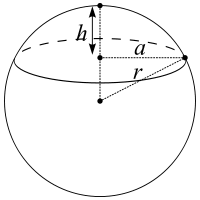The image above is a spherical cap.

To compute the volume of a spherical cap requires two essential parameters which are the radius of the base of the cap (a) and the height (h).

The formula for calculating the volume of the spherical cap:

V = πh(3a² + h²) ⁄ 6

Where;
V = Volume of the spherical cap
a = Radius of the base of the cap
h = Height of the spherical cap

Lets solve an example;
Find the volume of a spherical cap when the radius of the base is 12 cm and the height is 22 cm.

This implies that;
a = Radius of the base of the cap = 12 cm
h = Height of the spherical cap = 22 cm

V = πh(3a² + h²) ⁄ 6
V = 3.142 (22)(3(12)² + 22²) ⁄ 6
V = 3.142 (22)(3(144) + 484) ⁄ 6
V = 3.142 (22)(432 + 484) ⁄ 6
V = 3.142 (22)(916) ⁄ 6
V = 3.142 (20152)6
V = 63309.37 ⁄ 6
V = 10551.56

Therefore, the volume of the spherical cap is 10551.56 cm2.

## How to Calculate and Solve for the Total Surface Area of a Conical Frustum | The Calculator Encyclopedia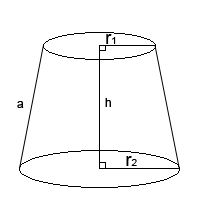The image above is a conical frustum.

To compute the total surface area of a conical frustum requires three essential parameters which are the radius of the lower base (R), radius of the upper base (r) and the height (h).

The formula for calculating the total surface area of a conical frustum;

A = π[R² + r² + (R + r)√((R – r)² + h²)]

Where;
A = Total surface area of the conical frustum
R = Radius of the lower base
r = Radius of the upper base
h = Height of the conical frustum

Let’s solve an example;
Find the total surface area of the conical frustum when the radius of the upper base is 11 cm, radius of the lower base is 17 cm and the height is 30 cm.

This implies that;
r = Radius of the upper base = 11 cm
R = Radius of the lower base = 17 cm
h = Height of the conical frustum = 30 cm

A = π[R² + r² + (R + r)√((R – r)² + h²)]
A = π[17² + 11² + (17 + 11)√((17 – 11)² + 30²)]
A = π[289 + 121 + (28)√((6)² + 900)]
A = π[289 + 121 + (28)√(36+ 900)]
A = π[289 + 121 + (28)√(936)]
A = π[289 + 121 + (28)(30.59)]
A = π[289 + 121 + 856.63]
A = π[1266.63]
A = 3979.25

Therefore, the total surface area of the conical frustum is 3979.25 cm².